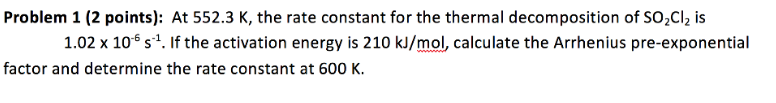# Problem: At 552.3 K, the rate constant for the thermal decomposition of SO 2Cl2 is 1.02 x 10-6 s-1. If the activation energy is 210 kJ/mol, calculate the Arrhenius pre-exponential factor and determine the rate constant at 600 K.

###### FREE Expert Solution
81% (190 ratings)###### Problem Details

At 552.3 K, the rate constant for the thermal decomposition of SO 2Cl2 is 1.02 x 10-6 s-1. If the activation energy is 210 kJ/mol, calculate the Arrhenius pre-exponential factor and determine the rate constant at 600 K.